# 遞迴 (Recursion)

`使用相同的方法，解決重複性的問題 (Recurrent Problems)。`

[e.g., 使用 迴圈 (loop)、判斷式 (decision)、賦值/指派 (assignment) ]

## 範例 —— int 陣列加總

``int a[] = {15, 37, 26, 9}; // 欲計算的陣列``

### 以 迴圈尋訪 並且加總

``````int sum(int[] a, int n) {
int result = 0; // 欲傳回之結果

if (n < 1)
throw new IllegalArgumentException("n should be >= 1");
else {
for (int i = 0; i < n; i++) // 用 for 迴圈 尋訪陣列
result = result + a[i]; // 將陣列值一一加總
}

return result; // 回傳結果
}``````

### 直接線性式遞迴 int 陣列加總

``````int sum(int[] a, int n) {
if (n < 1) // 陣列索引數不得小於 1
throw new IllegalArgumentException("n should be >= 1");
else if (n == 1) // 陣列索引數等於 1，返回陣列的 第 "1" 個元素
return a;
else // 否則，將問題拆解成 sum(a, n - 1) + a[n - 1]
return sum(a, n - 1) + a[n - 1];
}``````

## 種類 —— 依 呼叫方式

• 直接遞迴 (direct recursion)
• 間接遞迴 (indirect recursion)

### 直接遞迴 (direct recursion)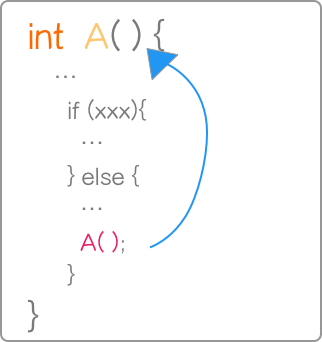### 間接遞迴 (indirect recursion)## 種類 —— 依 呼叫次數

• 線性遞迴 (linear recursion)
• 二元遞迴 (binary recursion)
• 多元遞迴 (multiple recursion)

### 線性遞迴 (linear recursion)### 二元遞迴 (binary recursion)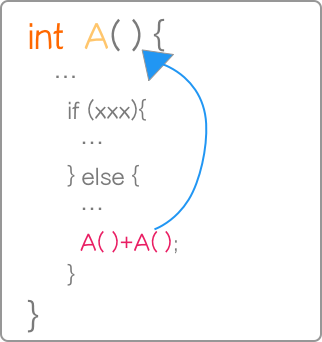## 種類 —— 尾端遞迴 (tail recursion)

• 直接遞迴 (direct recursion)
• 線性遞迴 (linear recursion)
• 且該 函式執行的最後一件事 為遞迴呼叫，且 只能是遞迴呼叫

### 尾端遞迴之重要性

#### 函式呼叫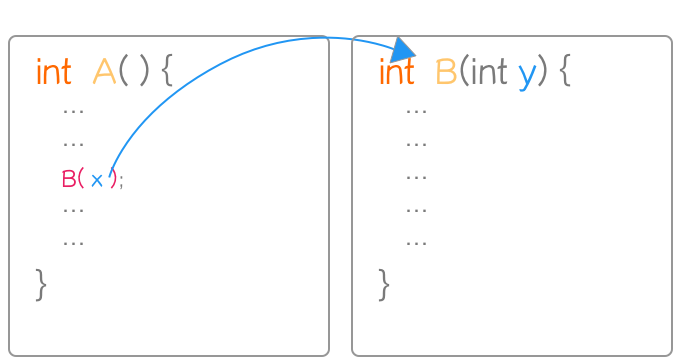1. 程式會建立一個 被稱為 活動紀錄 (activation record)堆疊框 (stack frame) 的結構，
保存 Function A ( ) 目前的執行狀態，並將其資訊 push 到 系統堆疊 (System Stack) 頂端，
2. 控制權轉移，且做參數傳遞 (譬如 上圖的 x 與 y)。
3. 執行 Function B( ) 。
4. Function B( ) 執行完成，做判斷:
``````if (Stack != empty) {
pop stack; // 取出參數、區域變數、返回位置 (return address)
依 返回位置 返回 並執行;
} ``````

—– 覺得很重要 o.o

### 遞迴/迭代轉換

`1 + 2 + 3 + 4 + 5 + ... + (n-1) + n`

#### 使用 迭代

``````int sum(int n) {

int result = 0;

for (int i = 1; i <= n; i++)
result = result + i;

return result;
}``````

#### 使用 遞迴 (線性 非尾端)

``````int sum(int n) {
if (n < 0)
throw new IllegalArgumentException("n should be >= 0");
else if (n == 0)
return 0;
else
return sum(n - 1) + n;
}``````

#### 使用 遞迴 (尾端)

``````public int sum(int n, int total) {
if (n == 0)
else
return sum(n - 1, total + n);
}``````

n 是 想要加總的數， total 就像是 上方非遞迴中的 區域變數一樣 (初始值 = 0)，用來儲存累計總和，

sum(10, 0) 問題會依序被分解為:
sum(9, 10);
sum(8, 19);
sum(7, 27);

``sum(n - 1, total + n);``

n – 1，不就是每次 迴圈 的 變化量嗎?
total + n 不就是 區域變數累加 ?

「上底加下底乘以高除以二」，勿捨本著墨 !

## 遞迴 vs. 非遞迴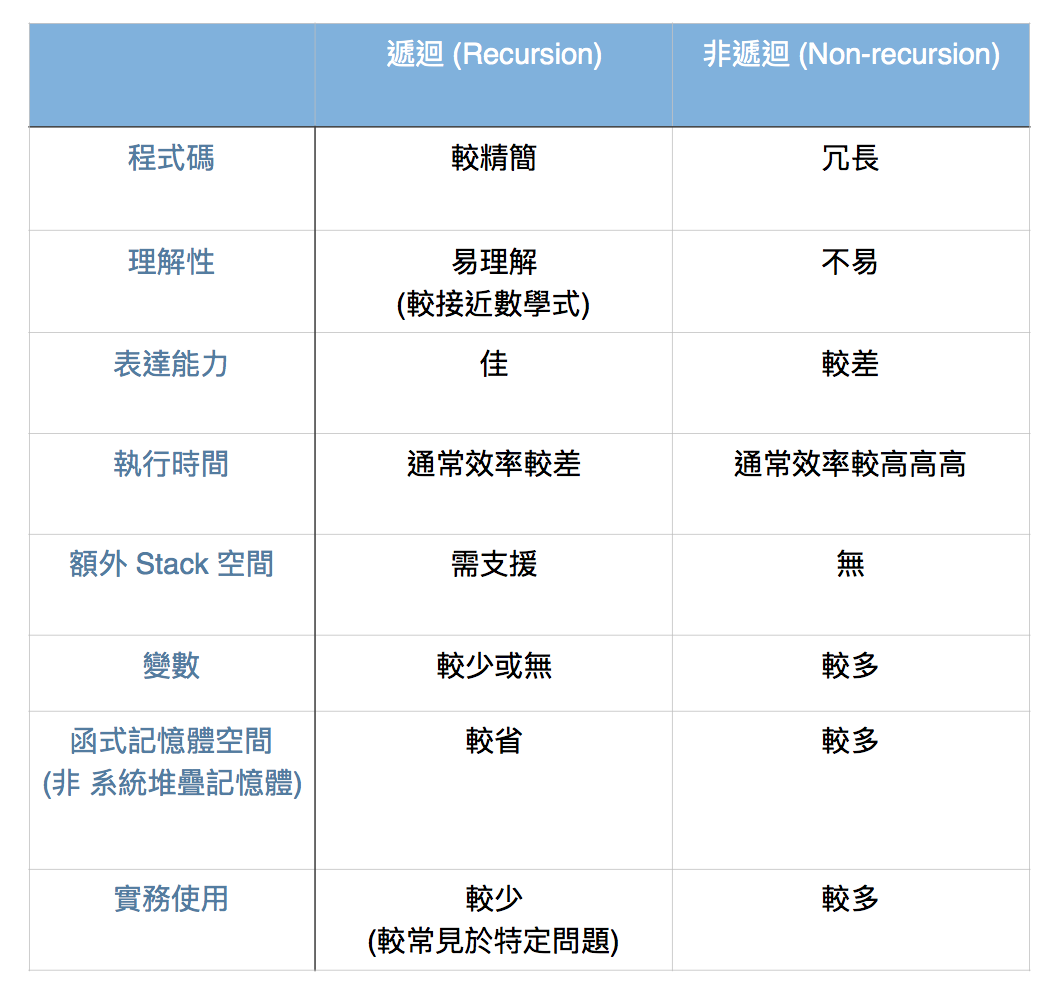## 遞迴 經典範例 —— (I) 費式數列 (Fibonacci Number)

Def:

F0 = 0
F1 = 1
Fn = Fn-1 + Fn-2 (n >= 2)

 F0 F1 F2 F3 F4 F5 F6 F7 F8 F9 F10 F11 F12 F13 F14 F15 F16 F17 F18 F19 F20 0 1 1 2 3 5 8 13 21 34 55 89 144 233 377 610 987 1597 2584 4181 6765

### 使用 遞迴 (二元 非尾端)

``````int fib(int n) {
if (n == 0)
return 0;
else if (n == 1)
return 1;
else
return fib(n - 1) + fib(n - 2);
}``````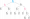### 使用 迭代

``````int fib(int n) {
if (n == 0)
return 0;
else if (n == 1)
return 1;
else {
// a: F(n-2)
// b: F(n-1)
int a = 0, b = 1, result = 0;

for (int i = 2; i <= n; i++) {
result = a + b;
a = b;
b = result;
}

return result;
}
}``````

### 使用 遞迴 (尾端)

``````public int fib(int n) {
return fib(n, 0, 1);
}

private int fib(int n, int a, int b) {
if (n == 0) return a;
return fib(n - 1, b, a + b);
}``````

### 使用 迭代 (Fast Doubling)F(2n) = F(n) [2F(n + 1) − F(n)]
F(2n + 1) = F(n + 1)2 + F(n)2

``````int fib(int n) {
int a = 0; // F(k)
int b = 1; // F(k + 1)

for (int bit = Integer.highestOneBit(n); bit != 0; bit >>>= 1) {
// Double it
int c = a * ((b << 1) - (a)); // F(2k)
int d = (a * a) + (b * b); // F(2k + 1)

if ((n & bit) != 0) {
int e = c + d; // F(2k + 2)
a = d; // k = 2k + 1
b = e;
} else {
a = c; // k = 2k
b = d;
}
}

// current k == n
return a;
}``````

### 使用 遞迴 (尾端 + Fast Doubling)

``````public int fib(int n) {
return fib(n, 0, 1, Integer.highestOneBit(n));
}

/*
* @param a -- F(k)
* @param b -- F(k + 1)
*/
private int fib(int n, int a, int b, int bit) {
if (bit == 0) return a;
// F(2k) = F(k) * [2 * F(k + 1) - F(k)]
int c = a * ((b << 1) - (a));
// F(2k + 1) = F(k)^2 + F(k + 1)^2
int d = (a * a) + (b * b);

if ((n & bit) != 0) {
int e = c + d; // F(2k + 2)
a = d; // k = 2k + 1
b = e;
} else {
a = c; // k = 2k
b = d;
}

bit >>>= 1;
return fib(n, a, b, bit);
}``````

## 遞迴 經典範例 —— (II) 河內塔 (Hanoi Tower)

Def:

• 一次只能搬移一個 disk
• 搬移過程中，小盤不可在大盤之下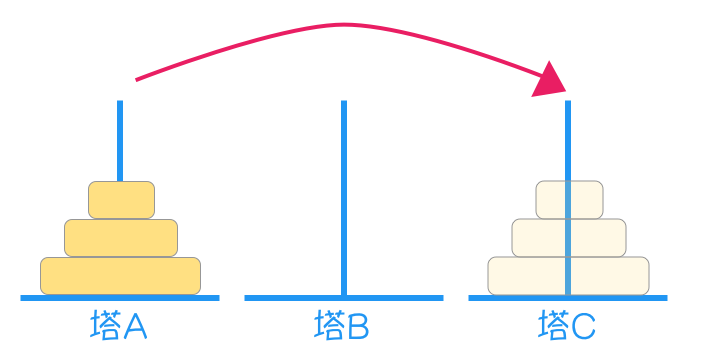### 解法邏輯 (尋找中繼塔):

1. 先將 塔A 上面的 n-1 個 盤子，移動到塔B (中繼為 c)
2. 將最大的盤子，搬至目的地 塔C
3. 將 塔B 剩下的盤子，搬移至目的地 塔C (中繼為 a)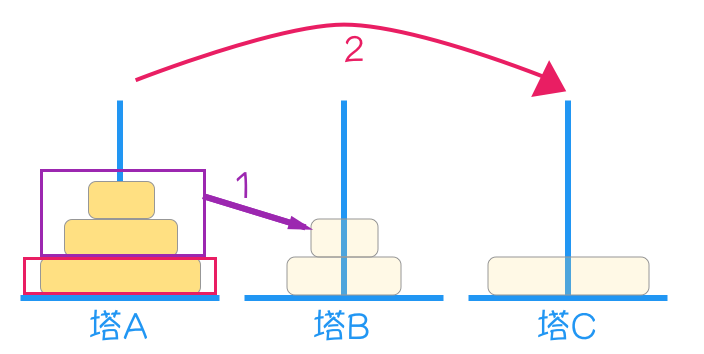Q: 『尛，不對啊，第二第三點聽起來很有道理，

• 先將 塔A 上面的 n-2 個 盤子，移動到塔B (中繼為 c)
• 將最大的盤子，搬至目的地 塔C
• 將 塔B 剩下的盤子，搬移至目的地 塔C (中繼為 a)

``````/**
* 計算河內塔
*
* @param n 搬移的盤子數目
* @param a 來源地 (source)
* @param b 中繼站 (bridge)
* @param c 目的地 (destination)
* @return
*/
void hanoi(int n, String a, String b, String c) {
if (n == 1) { // 只有一個盤子
System.out.println("將盤子從" + a + " 搬到" + c);
} else {
hanoi(n - 1, a, c, b); // 先搬 n-1 個盤子 到 b (將 c 當中繼)
hanoi(1, a, b, c); // 將剩下的一個盤子 由 a 搬到 c
hanoi(n - 1, b, a, c); // 將剛剛 塔b 的 n-1 個盤子 再由 b 搬到 c (a 當中繼)
}
}``````

```Result:

## 撰寫遞迴

(為大神 Max Howel 默哀 3 秒)#### 在《遞迴 (Recursion)》中有 8 則留言

1.匿名訪客表示:

間接遞迴標題那邊英文應該是indirect recursion. 寫得非常好!!

1.鄭 中勝表示:

已修正!!
非常感謝 😭 世界就是需要您這種人

2.吳建儒四資工三甲表示:

受益匪淺

1.鄭 中勝表示:

感謝吳同學 😁

3.匿名訪客表示:

溫習Tail Recusion時搜尋到這篇，寫的真的很淺顯易懂，非常感謝！

1.鄭 中勝表示:

謝謝您！ 之後將再升級內容喔 😆

4.彭子桓表示:

受用無窮